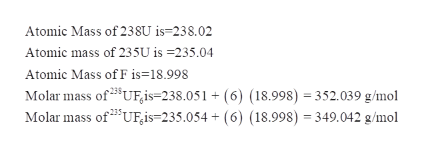# Uranium-235 can be separated from U-238 by fluorinating the uranium to form UF6 (which is a gas) and then taking advantage of the different rates of effusion and diffusion for compounds containing the two isotopes.Calculate the ratio of effusion rates for 238UF6 and 235UF6. The atomic mass of U-235 is 235.054 amu and that of U-238 is 238.051 amu.

Question
24 views

Uranium-235 can be separated from U-238 by fluorinating the uranium to form UF6 (which is a gas) and then taking advantage of the different rates of effusion and diffusion for compounds containing the two isotopes.

Calculate the ratio of effusion rates for 238UF6 and 235UF6. The atomic mass of U-235 is 235.054 amu and that of U-238 is 238.051 amu.

check_circle

Step 1

The ratio of the effusion rates of two gases:

Step 2

Calculation of molar mass of 238UF6 and 235...help_outlineImage TranscriptioncloseAtomic Mass of 23SU is=238.02 Atomic mass of 235U is -235.04 Atomic Mass of F is=18.998 Molar mass of3UF,is=238.051 + (6) (18.998) 352.039 g/mol Molar mass of23 UF,is=235.054 + (6) (18.998) 349.042 g/mol fullscreen

### Want to see the full answer?

See Solution

#### Want to see this answer and more?

Solutions are written by subject experts who are available 24/7. Questions are typically answered within 1 hour.*

See Solution
*Response times may vary by subject and question.
Tagged in

### Physical Chemistry